# 基于MATLAB的Rossler系统随参数变化的研究

1、引言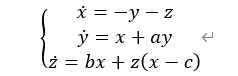x’，y’和z’表示对自变量时间t求导数。(x，y，z)表示系统的状态。a，b，c，是系统参数。方程看上去很简单，除去第三个方程中的二次项zx，则系统是线性的。但事实是，这个简单系统存在混沌行为。

2、对方程的定性分析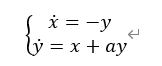3、参数对方程的影响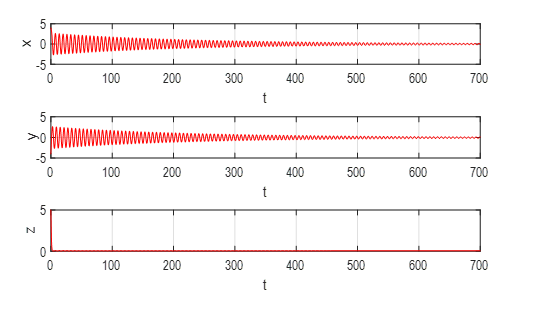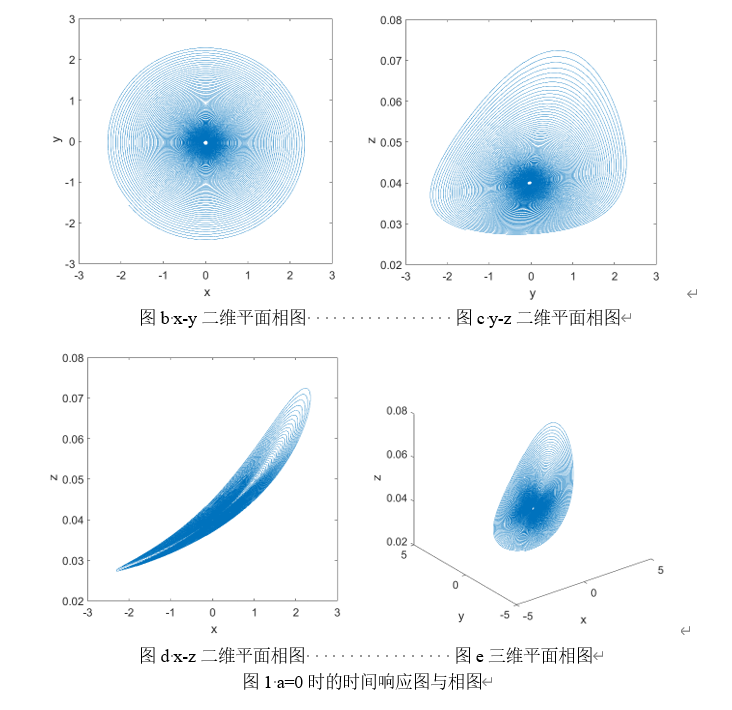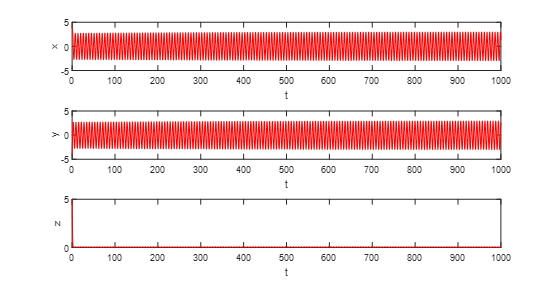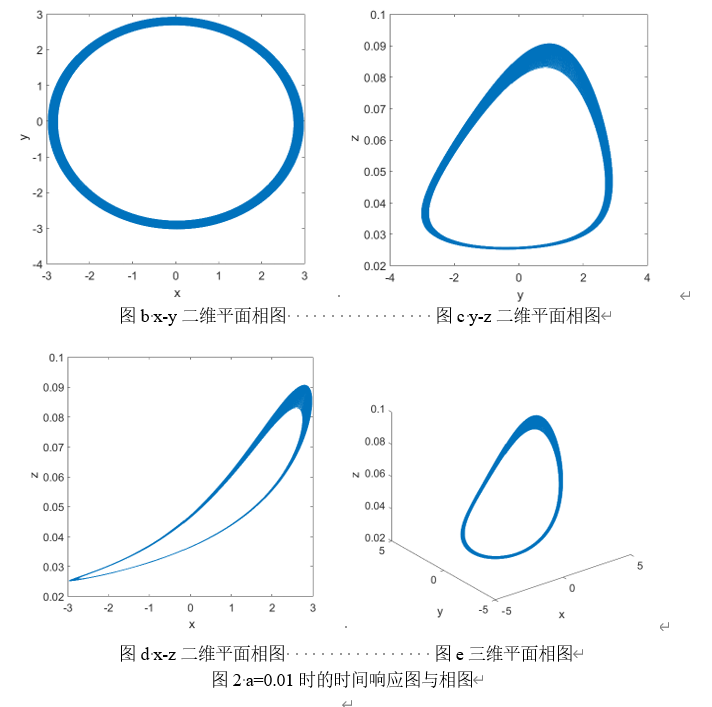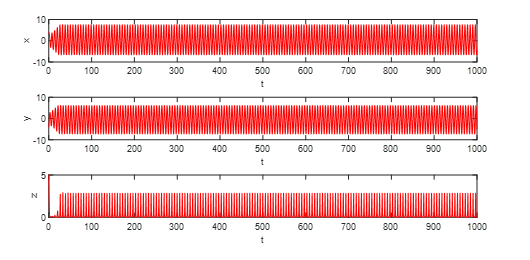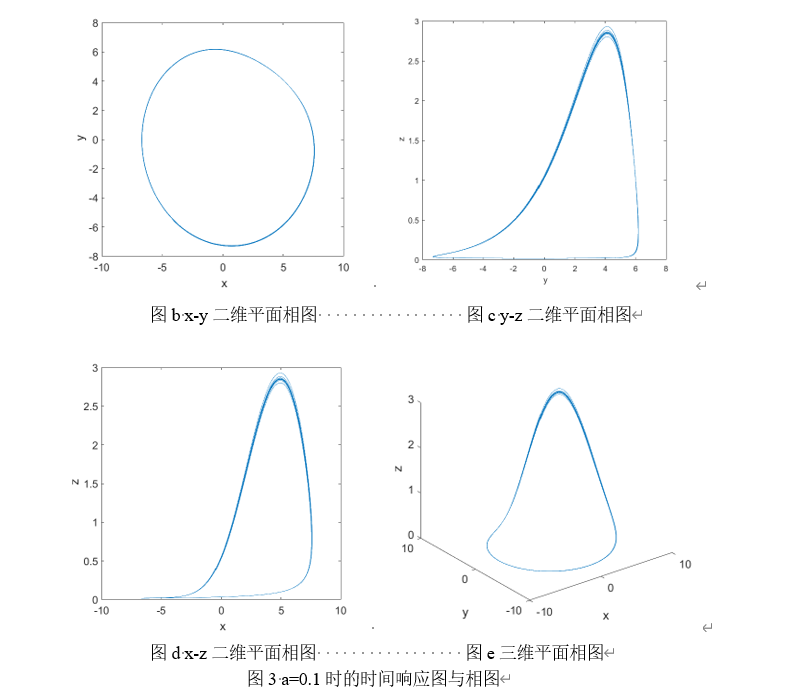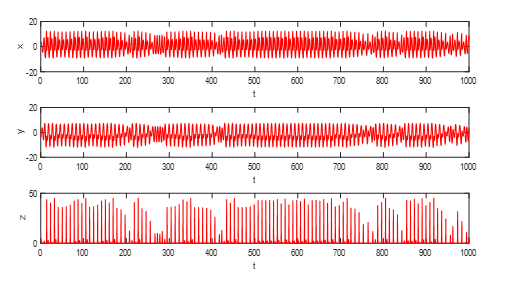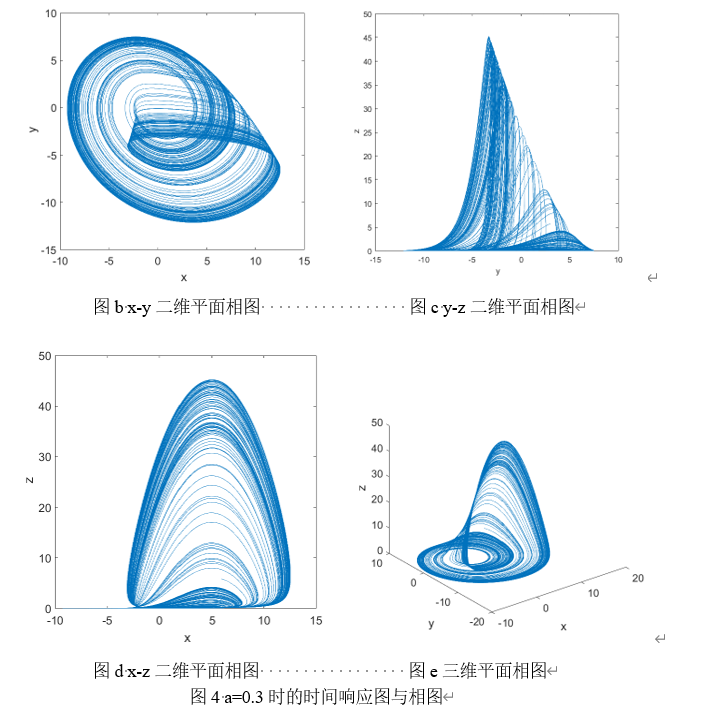4、倍周期分岔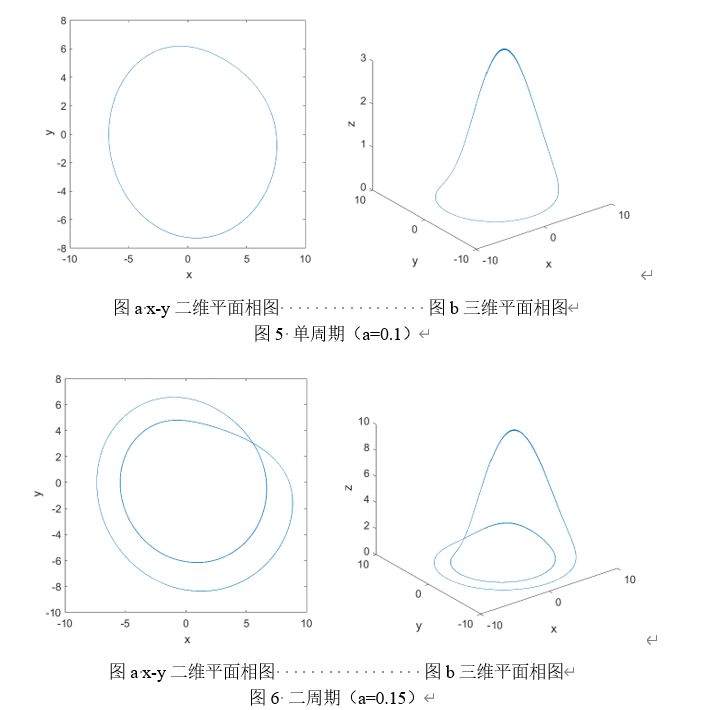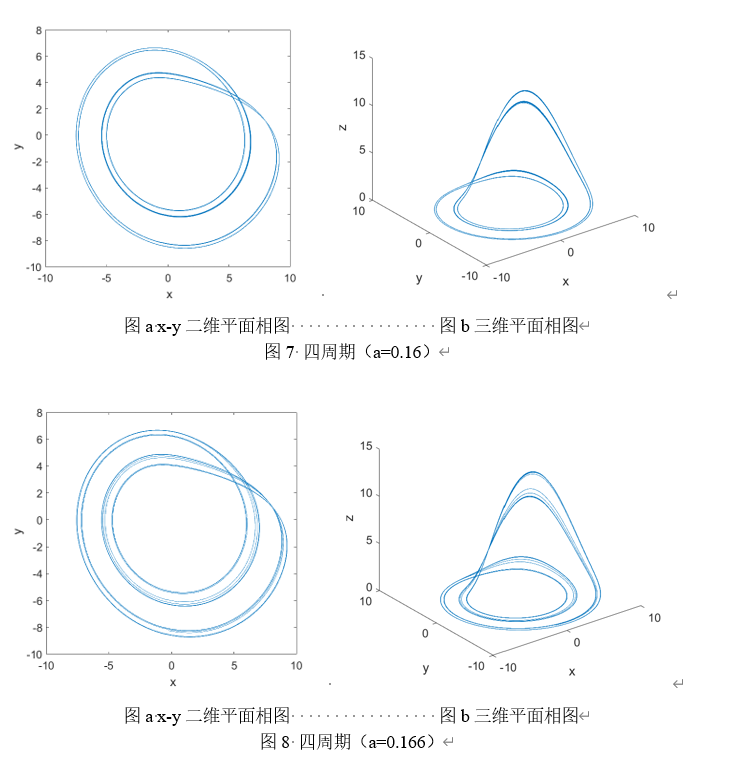5、结论

（1）在混沌态Rossler系统的相图曲线对初值高度敏感，其行为不可预测，呈现随机性，即在长时间运动后一个确定的运动变成无法预言的随机行为。这种对初始条件的敏感性和对未来的无法预见性，是混沌解的显著特征。
（2）随着参数的变化，Rossler系统通过倍周期分岔逐步走向混沌。
（3）在混沌态，Rossler系统的相轨迹局部不稳定，但全局稳定，相轨迹始终被限制在相图中的某一区域。表现出典型的吸引子特征。混沌系统看似无序。却有着内在的深层次规律性。

6、程序代码

（1）rossler.m

function dy=rossler(t,y)
%%rossler
a=0.3;b=0.2;c=5.0;
dy=[-(y(2)+y(3));y(1)+a*y(2);b+y(1)*y(3)-c*y(3)];
end


（2）ross.m

x0=[4;-4;5];
tspan=[0:0.001:1000];
[T,X]=ode45('rossler',tspan,x0);
figure(1);
subplot(3,1,1),plot(T,X(:,1),'r');
xlabel('t');
ylabel('x');
subplot(3,1,2),plot(T,X(:,2),'r');
xlabel('t');
ylabel('y');
subplot(3,1,3),plot(T,X(:,3),'r');
xlabel('t');
ylabel('z');

figure(2);
plot(X(30000:end,1),X(30000:end,2));%grid;
xlabel(' x');
ylabel(' y');

figure(3);
plot(X(30000:end,1),X(30000:end,3));%grid;
xlabel(' x');
ylabel(' z');

figure(4);
plot(X(30000:end,2),X(30000:end,3));%grid;
xlabel(' y');
ylabel(' z');

figure(5);
plot3(X(30000:end,1),X(30000:end,2),X(30000:end,3));%grid;
xlabel(' x');
ylabel(' y');
zlabel(' z');


［1］黄润生，黄浩，编著.混沌及其应用[M].武汉大学出版社，2005.
［2］王晓艳.非线性混沌电路的分析与设计[D].哈尔滨工程大学，2010.
［3］刘 锋,穆肇骊,邱祖廉.Rossler混沌系统脉冲同步[J].物理学报,1999,48(7):1198-1204

04-0403-1794
10-1162
08-23601
04-021787
03-16146
12-04
03-16
05-03
03-16221
06-08点击重新获取扫码支付余额充值